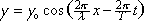For a medium with a continuous traveling wave in it, this equation gives the displacement of each point in the medium, from that point's equilibrium position, as a function of position along the length of the medium and as a function of time.

 y is the displacement of the point at distance x from the origin, from its equilibrium position, at time t. y is a variable depending on both position x along the length of the medium, and time. yo (to be read y-max rather than y-zero) is the maximum displacement any point in the medium has from its equilibrium position. yo is an upper limit established by the oscillator that is causing the waves. As such, yo is a constant. yo is the amplitude of the wave. lambda is the wavelength of the wave. It is the distance, at any instant in time, along the length of the medium, over which the wave pattern repeats itself once. Lambda is a constant. x is the position measured along the length of the medium from a specified origin, e.g. from the oscillator that is causing the wave. x is a variable. T is the period of oscillations. Each point in the medium undergoes simple harmonic motion about its own equilibrium position. T is the period of that simple harmonic motion. T is a constant. t is time. t is a variable.

If one focuses one's attention on a part of a part of a wave, the peak of a crest for instance, one sees that part of the wave move along the medium. For that part of the wave, in order for it to remain the part of the wave that it is, the argument of the cosine must remain a constant. In order for the argument of the cosine, as written, to remain a constant, as time t increases, x must increase. If x is increasing with time, the part of the wave under consideration, and thus the entire wave, must be moving in the positive x direction. In cases where there is a plus sign between the two terms making up the argument of the cosine function, x must decrease with increasing t to keep the argument of the cosine constant. This would be the case for a wave moving in the negative-x direction.